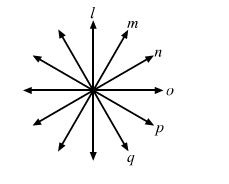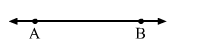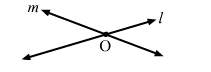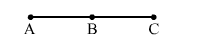# How many lines can be drawn through a given point?

Question:

(i) How many lines can be drawn through a given point?
(ii) How many lines can be drawn through two given points?
(iii) At how many points can two lines at the most intersect?
(iv) If Aand C are three collinear points, name all the line segments determined by them

Solution:

(i) Infinite lines can be drawn through a given point.(ii) Only one line can be drawn through two given points.(iii)  At most two lines can intersect at one point.(iv) The line segments determined by three collinear points A, B and C are

$\overline{A B}, \overline{B C}$ and $\overline{A C}$.